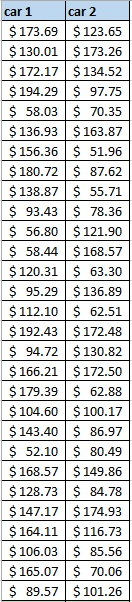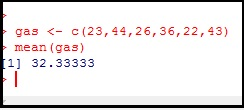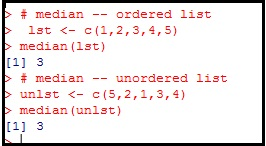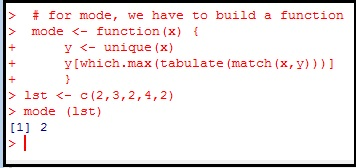# R: Intro to Statistics – Central Tendency

## Central Tendency

One of the primary purposes of statistics is to find a way to summarize data. To do so, we often look for numbers known as collectively as measurements of central tendency (mean, median,  mode).

Look at the list below. This is a list of weekly gas expenditures for two vehicles. Can you tell me if one is better than the other, or are they both about the same?How about if I show you this?Using the average, you can clearly see car2 is more cost efficient. At approx \$21 a week, that is a savings of \$630 over the course of the 30 weeks in the chart. That is a big difference, and one that is easy to see. We can see this using one of the 3 main measures of central tendency – the arithmetic mean – popularly called the average.

## Mean

The mean – more accurately the arithmetic mean – is calculated by adding up the elements  in a list and dividing by the number of elements in the list.In R, finding a mean is simple. Just put the values you want to average into a vector (**note to make a vector in R: var  <-c(x,y,z)) We then put the vector through the function mean()## Median

The median means, simply enough, the middle of an ordered list. This is also easy to find using R. As you can see, you do not even need to sort the list numerically first. Just feed the vector to the function median()## Mode

This is the last of 3 main measure. Mode returns the most common value found in a list. In the list 2,3,2,4,2 – the mode is 2. Unfortunately R does not have a built in mode function, so for this, we will have build our own function.

For those familiar with functions in programming, this shouldn’t be too foreign of a concept. However, if you don’t understand functions yet, don’t fret. We will get to them soon enough. For now, just read over the code and see if you can figure any of it out for yourself.